﻿ Derivations and Integrations on RingsPublications are Open
Access in this journal
Article Versions
Export Article
• Normal Style
• MLA Style
• APA Style
• Chicago Style
Research Article
Open Access Peer-reviewed

### Derivations and Integrations on Rings

Michael Gr. Voskoglou
American Journal of Applied Mathematics and Statistics. 2019, 7(2), 75-78. DOI: 10.12691/ajams-7-2-4
Received December 10, 2018; Revised January 13, 2019; Accepted February 09, 2019

### Abstract

In this paper properties are studied of the differential ideals of a ring R and of the iterated skew polynomial rings over R defined with respect to a finite set of commuting derivations of R. The concept of the integration of R associated to a given derivation of R is also introduced and some funamental properties of it are studied. This new concept generalizes basic features of the indefinite integrals.

### 1. Introduction

All the rings considered in this paper are with identity and they have characteristic zero.

A derivation on a ring is a function which generalizes certain features of the traditional derivative operator. On the other hand the term integration is connected to the computation of an integral.

In the present work properties are studied of the differential ideals of a ring R and of the iterated skew polynomial rings over R defined with respect to a finite set of commuting derivations of R. The concept of the integration of R associated to a given derivation of R is also introduced and some fundamental properties of it are studied. This new concept generalizes basic features of the indefinite integrals.

The paper is organized as follows: The next section contains the information about derivations and the differential simplicity of a ring needed for the good understanding of the rest of the work, whereas the main results are presented in Section 3. The article closes with the conclusions and some hints for future research on the subject, which are contained in Section 4.

### 2. Derivations and Differential Simplicity of Rings

We start by recalling the following definitions:

2.1. Definition: Let R be a ring. Then a map

d: R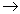R is called a derivation of R, if and only if, d(x+y) = d(x) + d(y) and d(xy) = xd(y) + d(x)y, for all x, y in R.

Observe that d(1) = d(1.1) = 2d(1), therefore d(1) = 0. The set of all derivations of R is denoted by DerR.

Given a non commutative ring R and an element s in R it is easy to check that the map d: RR defined by d(r) = sr-rs is a derivation of R, called the inner derivation of R induced by s. For distinguishing between the two cases, a derivation of R which is not inner is called an outer derivation.

2.2 Definition: Let R be a ring and let d be a derivation of R. Then an ideal I of R is said to be a d-ideal, if d(I)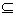I. If the only d-ideals of R are 0 and R, then R is called a d-simple ring and d is called a simple derivation of R.

Non commutative d-simple rings exist in abundance; for example every simple ring is d-simple for any derivation d of R. On the other hand, there is not known any general criterion under which one can decide whether or not a commutative ring possesses simple derivations. Typical examples of such rings are the polynomial rings in finitely many variables over a field 1 and the regular local rings of finitely generated type over a field 2. More examples can be found in 1, whereas in 3 geometrical examples are presented of smooth varieties (algebraic sets) over a field with coordinate rings possessing simple derivations.

It is well known that if a commutative ring R is d-simple then R is an integral domain and also that if R has no non zero prime d-ideals, then R is a d-simple ring ( 4; Corollary 1.5).

Definition 2.2 can be generalized for a finite set D of derivations of R as follows:

2.3 Definition: Let D be a finite set of derivations of R. Then an ideal I of R is called a D-ideal if d(I)I for all d in D and R is called a D-simple ring, if it has no proper non zero D-ideals (differential simplicity of R).

Obviously, if R is a d-simple ring for some d in D, then R is also a D-simple ring, but the converse is not true; e.g. this happens with the coordinate ring of the real sphere ( 5, Lemma 3.1).

2.4 Definition: Let R be a ring and let d be a derivation of R. Define on the set S of all polynomials in one variable x over R addition in the usual way and multiplication by the rule: xr=rx+d(r), for all r in R. It is well known then that S becomes a non commutative ring denoted by R[x, d] and called a skew polynomial ring (of derivation type) over R (e.g. see 6, p.35).

Such rings, which are also known as Ore extensions, have been firstly introduced by O. Ore 7 to be used as counter examples. Note that skew polynomial rings can also be defined over R with respect to an endomorphism f of R and in a more general context with respect to f and an f-derivation d of R 6, which is a generalization of the concept of the ordinary derivation.

We continue with the following useful Lemma:

2.5 Lemma: Let R be a ring, let d be a derivation of R and let S=R[x, d] be the corresponding skew polynomial ring over R. Let also d* be another derivation of R. Then d* extends to a derivation of S by d*(x)=0, if, and only if, d* commutes with d.

Proof 8: Obviously d* extends to a derivation of S, if, and only if, d*(x) can be defined in a way compatible to multiplication in S. In other words, if d*(x)=h, then for all r in R we must have d*(xr)= d*(rx)+d*[d(r)]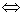xd*(r)+hr=rh+d*(r)x+d*[d(r)]d*(r)x+ d[d*(r)]+hr= rh+d*(r)x+d*[d(r)],

Therefore h=0d[d*(r)]= d*[d(r)], which completes the proof.

Let now D = {d1, d2,…,dn} be a finite set of derivations of R commuting to each other; i.e. we have that di o dj=dj o di, i, j = 1, 2,…,n. Consider the set Sn of all polynomials in n variables x1, x2, …,xn and define addition in Sn in the usual way and multiplication by the rules xir = rxi+di(r), xixj = xjxi, for all r in R and all i, j = 1,2,…,n.

Set S1=R[x1, d1] and, using Lemma 2.5, consider the skew polynomial rings S2=S1[x2, d2],…., Sk+1=Sk[xk, dk],…, Sn=Sn-1[xn, dn].

The ring Sn=R[x1, d1][x2, d2]….,[xn, dn], introduced by Voskoglou 8, is called an iterated skew polynomial ring (ISPR) of derivation type over R and for brevity will be denoted by Sn=R[X, D].

Voskoglou 9 has also introduced ISPRs over R with respect to a finite set {f1,f2,…,fn}of monomorhisms of R and a corresponding set {d1, d2, …, dn} of fi-derivations of R, such that di o dj=dj o di, di o fj= fj o di and fi o fj = fj o fi.

Other types of ISPRs, in which multiplication is defined only by the rule xir = rxi+di(r), for all r in R and all i=1,2,…,n, but the variables need not to commute, have been introduced by Kishimoto 10 and by others. To distinguish between the two cases we denote the ISPRs of the second case by Sn*.

Note that in Sn* the derivations of D need not commute to each other. We prove the following result about this:

2.6 Proposition: Let R be a ring and let D be a finite set of derivations of R. Then, if the variables of an ISPR over R defined with respect to D commute, the derivations of D commute too.

Proof: Given r in R and two variables xi and xj of the ISPR over R we have that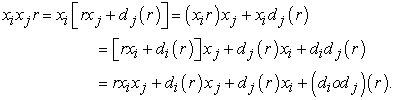In the same way we find that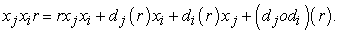Assuming that xixj = xjxi the result follows by equating the right members of the last two equations.

The ISPRs have found recently two important applications resulting to the renewal of the researchers’ interest about them. The former concerns the ascertainment that many Quantum Groups (i.e. Hopf algebras having in addition a structure analogous to that of a Lee group 11), which are used as a basic tool in Theoretical Physics, can be expressed and studied in the form of an ISPR. The latter concerns the utilization of ISPRs in Cryptography for analyzing the structure of certain codes 12.

Voskoglou has also proved the following result 8:

2.7 Theorem: Let R be a ring, let D = {d1, , …, dn} be a finite set of derivations of R commuting to each other and let Sn=R[X, D] be the corresponding ISPR over R. Assume further that di is an outer derivation of Si-1, where So = R. Then Sn is a simple ring, if, and only if, R is a D-simple ring.

As an example, consider the polynomial ring R=k[y1, y2,…,yn] over a field k and the set D={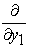,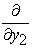,…,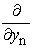} of partial derivatives of R. Then it is straightforward to check that R is a D-simple ring ( 13; Example 1), therefore by the previous theorem the ISPR R[X, D] is a simple ring.

Theorem 2.6 for n=1 is due to D. Jordan 14

The following definition generalizes the notion of a prime ideal of a ring:

2.8 Definition: Let R be a ring and let D be a finite set of derivations of R. Then a D-ideal I of R is said to be a D-prime ideal, if given any two D-ideals A and B of R such that ABI, it is either AI or BI. In particular, R is called a D-prime ring, if (0) is a D-prime ideal of R.

The next result 15 establishes a relationship among the prime ideals of Sn and the D-prime ideals of R:

2.9 Theorem: Let R be a ring, let D be a finite set of derivations of R commuting to each other and let Sn=R[X, D] be the corresponding ISPR over R. Then:

Ÿ If P is a prime ideal of Sn, P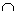R is a D-prime ideal of R.

Ÿ If I is a D-prime ideal of R, ISn is a prime ideal of Sn.

### 3. Main Results

Let R be a commutative ring, let d be a derivation and let I be an ideal of R, Then it is straightforward to check that d-1(I) = {r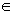R: d(r)I}is a subring of R. We shall prove the following result:

3.1 Theorem: Let P be a prime d-ideal of R, then the ring d-1(P) is integrally closed in R.

Proof: It suffices to show that, if r is an element of R integral over d-1(P), then r is in d-1(P).

In fact, since r is integral over d-1(P), there exists a monic polynomial f(x) = xn+an-1xn-1+ … +a1x+ao of minimal degree n with coefficients in d-1(P), such that f(r) = rn+an-1rn-1+ … +a1r+ao = 0. Differentiating this equation with respect to d one gets that

[nrn-1+(n-1)an-1rn-2+..+a1]d(r)+d(an-1)rn-1+..+d(a1)r = 0 or rod(r) = -[d(an-1)rn-1+..+d(a1)r], with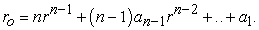(1)

But, since an-1, ..,a1 are in d-1(P), we get that d(an-1), .., d(a1) are in P. Therefore rod(r) is in P, which implies that either ro is in P or d(r) is in P. But, if ro is in P, d(ro) is also in P, therefore ro is in d-1(P). Thus equation (1) contradicts to the minimality of n in f(x). Consequently d(r) is in P, which shows that r is in d-1(P) and this completes the proof of the theorem.

Let now s = a+d(b) be an element of I + d(I), with a, b in the ideal I of R. Then d(rb)=rd(b)+d(r)b, therefore rs = ra+rd(b) = ra+[d(rb)-d(r)b]=[ra-d(r)b]+d(rb) is in I+d(I), for all r in R. Consequently I + d(I) is an ideal of R.

Assume now that R is a local ring, i.e. a Noetherian ring with a unique maximal ideal M. If M is not a d-ideal of R, then M+d(M) is an ideal of R containing properly M, therefore M+d(M)=R. On the other hand, it becomes clear that the ideal Mk+d(Mk)M, for all integers k, k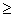2. In particular, for k=2 we shall prove the following result:

3.2 Theorem: Let R be a local ring with maximal ideal M and let d be a derivation of R such that M is not a d-ideal of M. Then M2+d(M2)=M.

Proof: Since R is a Noetherian ring, M is a finitely generated ideal of R. Therefore, we can write M=(m1, m2, …, mk), for some positive integer k.

Since M is not a d-ideal of R, there exists at least one generator ms of M such that d(ms) is not in M. We can write then M=(m1+ms, m2+ms, …, mk+ms). Therefore, without loss of generality we may assume that d(mi) is not in M, for all i=1, 2, …, k. Consequently d(mi) is a unit of R, because otherwise we should have that (d(mi)) is a proper ideal of R, which implies that (d(mi))M, or d(mi)M, a contradiction. In other words, there exists ri in R such that rid(mi)=1.

Then d(mi2)=2mid(mi)=2mi(ri-1) is in M2+d(M2), therefore mi =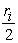[2mi(ri-1)] is also in M2+d(M2), which completes the proof.

We now introduce the following concept:

3.3 Definition: Let R be a ring and let d be in DerR. Then the integration of R associated to d is a map i: RR such that d[i(x)] = x, for all x in R.

Next we shall prove:

3.4 Theorem: Let d be an injective derivation of a ring R and let i be the integration of R associated to d. Then i is a derivation of R, if, and only if,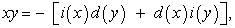for all x, y in R.

Proof: For all x, y in R we have by definition 2.2 that d[i(x+y)] = x+y. We also have that d[i(x)+i(y)] =d[i(x)]+d[i(y)]=x+y. Therefore, since d is an injective map, we obtain that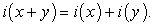(2)

On the other hand, we have that d[i(xy)]=xy and d[xi(y) + i(x)y] = d[xi(y)] + d[i(x)y] = x[d[i(y)] +d(x)i(y) + i(x)d(y) + d[i(x)]y =2xy+d(x)i(y)+i(x)d(y).

On comparing the last two equations we obtain that d[i(xy)]=d[xi(y)+i(x)y], if, and only if, xy=2xy+d(x)i(y) + i(x)d(y) .

This, combined to the fact that d is an injective map, it finally shows that i(xy)]=xi(y)+i(x)y, if, and only if,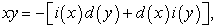(3)

which, together with equation (2) completes the proof of the theorem.

Theorem 3.4 has the following two important corollaries:

3.5 Corollary: Let R be a ring, let d be an injective outer derivation R and let i be the integration of R associated to d. Assume further that equation (3) holds for all x, y in R. Then:

The skew polynomial ring S=R[x, i] is simple, if, and only if, R is an i-simple ring.

If P is a prime ideal of S, PR is an i-prime ideal of R, whereas if I is an i-prime ideal of R, IS is a prime ideal of S.

Proof: 1) By Theorem 3.4 i is a derivation of R, therefore the result follows by applying Theorem 2.7 for n=1.

2) It turns out by combining Theorem 3.4 and Theorem 2.9 for n=1.

Next we need the following lemma:

3.6 Lemma: Let D = {d1, d2,…, dn} be a finite set of injective derivations of a ring R commuting to each other and let F = {f1, f2,…, fn} be the set of integrations of R, such that fi is associated to di, i=1,2,..,n. Then the integrations of F commute to each other.

Proof: Given r in R, we have that didj[fifj(r)] = djdi[fifj(r)] = dj[(difi)fj(r)] = dj[fj(r)] = r. In the same way it turns out that didj[fjfj(r)] = r, therefore didj[fifj(r)] = didj[fjfj(r)].

But the map didj is injective, hence fi o fj = fj o fi and the result follows.

3.7 Corollary: Let D = {d1, d2,…, dn} be a finite set of injective derivations of a ring R commuting to each other and let F = {f1, f2,…, fn} be the set of integrations of R, such that fi is associated to di, i=1,2,..,n. Assume further that for the derivation di and the associated to it integration fi the equation (3) holds for all the elements of Si-1 (where So=R). Then one can define the ISPR Sn = R[x, F], where we have:

Ÿ If P is a prime ideal of Sn, PR is a D-prime ideal of R.

Ÿ If I is a D-prime ideal of R, ISn is a prime ideal of Sn.

Proof: By Theorem 3.4 the elements of F are derivations of R and by Lemma 3.6 they commute to each other. Therefore we can define the ISPR Sn = R[x, F] and the result follows by Theorem 2.9

### 4. Discussion and Conclusions

In this work we studied properties of the differential ideals of a ring R and of the ISPRs of derivation type over R. The notion of an integration of R associated to a given derivation of R was also introduced and some fundamental properties of it were studied. This new concept generalizes basic features of the indefinite integrals and therefore a further research on its properties in connection to corresponding properties of the associated derivations seems to have its own importance.

For example, an open question is if the first case of Corollary 3.5 can be extended to ISPRs in finitely many variables defined as in Corollary 3.7. This could happen if each fi in F in Corollary 3.7 is an outer derivation of Si-1, but the conditions under which this could happen are under investigation.

### References

  Voskoglou, M. Gr., “Differential simplicity and dimension of a commutative ring”, Rivista Mathematica University of Parma, 6(4), 111-119, 2001. In article  Hart, R., “Derivations on regular local rings of finitely generated type”, Journal of London Mathematical Society, 10, 292-294. 1973. In article  Voskoglou, M. Gr., “A Study on Smooth Varieties with Differentially Simple Coordinate Rings”, International Journal of Mathematical and Computational Methods, 2, 53-59, 2017. In article  Lequain, Y., “Differential simplicity and complete integral closure, Pacific Journal of Mathematics, 36, 741-751, 1971. In article View Article  Voskoglou, M. Gr., “A note on the simplicity of skew polynomial rings of derivation type”, Acta Mathematica Universitatis Ostraviensis, 12, 61-64, 2004. In article  Cohn, P. M., Free Rings and their Relations, London Mathematical Society Monographs, Academic Press, 1974. In article  Ore, O., “Theory of non commutative polynomials”, Annals of Mathematics, 34, 480-508, 1933. In article View Article  Voskoglou, M. Gr., “Simple Skew Polynomial Rings”, Publications De L’Institut Mathematique, 37(51), 37-41, 1985. In article  Voskoglou, M. Gr., “Extending Derivations and Endomorphisms to Skew Polynomial Rings”, Publications De L’Institut Mathematique, 39(55), 79-82, 1986. In article  Kishimoto, K., “On Abelian extensions of rings I”, Mathematics Journal Okayama University, 14, 159-174, 1969-70. In article  Majid, S., “What is a Quantum group?”, Notices of the American Mathematical Society, 53, 30-31, 2006. In article  Lopez-Permouth, S., “Matrix Representations of Skew Polynomial Rings with Semisimple Coefficient Rings, Contemporary Mathematics, 480, 289-295, 2009. In article View Article  Voskoglou, M. Gr., “Derivations and Iterated Skew Polynomial Rings”, Internatinoal Journal of Applied Mathematics and Informatics, 5(2), 82-90, 2011. In article  Jordan, D., “Ore extensions and Jacobson rings”, Journal of London Mathematical Society, 10, 281-291, 1975. In article View Article  Voskoglou, M. Gr., “Prime ideals of skew polynomial rings”, Rivista Mathematica University of Parma, 4(15), 17-25 , 1989. In articleThis work is licensed under a Creative Commons Attribution 4.0 International License. To view a copy of this license, visit http://creativecommons.org/licenses/by/4.0/